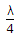# Electronics and Communication Engineering - Microwave Communication

### Exercise :: Microwave Communication - Section 8

36.

Dielectric constant and conductivity of rocky soil and flat sandy soil are around

 A. 10 and 20 mho/cm respectively B. 20 and 10 mho/cm respectively C. 2 and 5 mho/cm respectively D. 0.5 and 2 mho/cm respectively

Explanation:

No answer description available for this question. Let us discuss.

37.

The main advantage of using micro waves for communications is

 A. large bandwidth B. small bandwidth C. low power D. high power

Explanation:

No answer description available for this question. Let us discuss.

38.

A line of length less thanand open circuited at far end behaves as

 A. inductance L B. capacitance C C. L and C in series D. L and C in parallel

Explanation:

No answer description available for this question. Let us discuss.

39.

The beam of a radar should be

 A. very narrow B. very wide C. neither too narrow nor very wide D. either (b) or (c)

Explanation:

No answer description available for this question. Let us discuss.

40.

TE10 mode of propagation of electromagnetic energy in waveguides can be thought of as

 A. three TEM waves travelling along length of wave-guide B. One TEM wave travelling along length of wave guide and one TEM wave propagation in the transverse plane C. One TEM wave travelling along the length of wave guide and two TEM waves D. none of the above